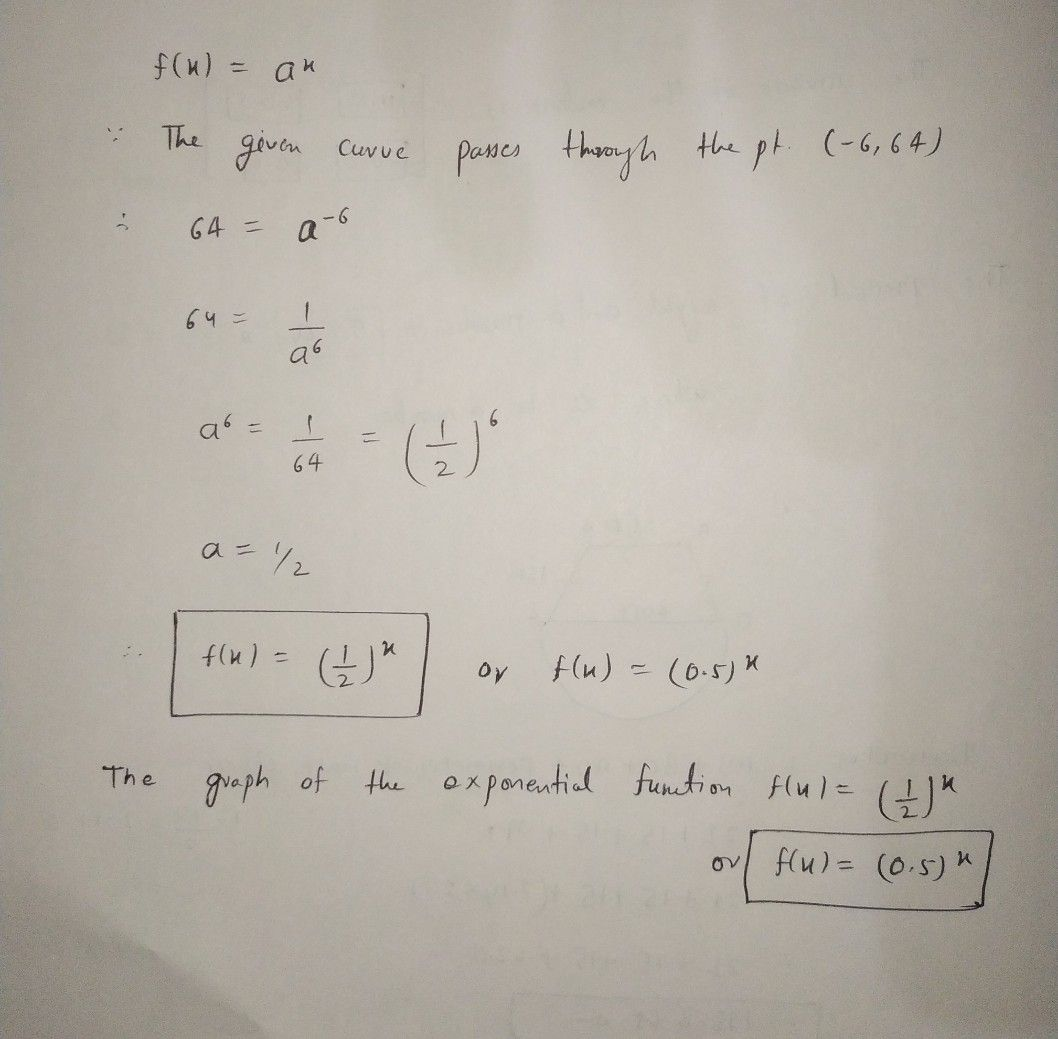Symbol
ProblemGive a rule of the $to\pi mf\left(x\right)=a^{x}$ to define the exponential function whose graph contains the given point. (a) $\left(2,16\right)$ (b) $\left(-6.64\right)$ $\left(a\right)$ The graph of the exponential function $f\left(x\right)=4^{x}$ passes through the point $\left(2,16\right)$ (Simplify your answer. Use integers or fractions for any numbers in the expression.) $\left(b\right)$ The graph of the exponential function $f\left(x\right)=\bar{1}$ pases through the point $\left(-6.64\right)$ (Simplify $y0∪r$ answer. Use integers or fractions for any numbers in the expression.)
Algebra
Search count: 106
SolutionQanda teacher - AskSubhashHey dear feel free to ask queries
please evaluate the answer if you satisfied
Please give 3000 coins as thank you gift this will not reduce your coins